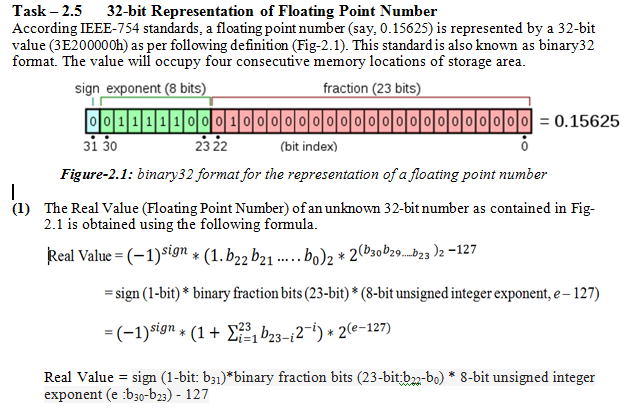# float variables modified my number....

I put a really long floating number to a float variable for my uno. What I expect was it will put zeros after 7 or 8 digits. But instead it put some other values there. Is this something expected?

``````float test1 = 0;

void setup() {
// put your setup code here, to run once:

test1 = 22.42394520349523423423;

Serial.begin(19200); // it is actually 9600 from external

}

void loop() {
// put your main code here, to run repeatedly:
Serial.println(test1,26);
delay(100);

}
``````

and I got::

22.42394447326660156250000000
22.42394447326660156250000000
22.42394447326660156250000000
22.42394447326660156250000000
22.42394447326660156250000000
22.42394447326660156250000000
22.42394447326660156250000000

Yeah, that's as expected. floats != decimal point. Floats are good to have a variable that can hold a very large number or a very small number with limited storage. That comes however with limited accuracy. On most standard Arduino's a float is 32-bit will give you an accuracy of around 6 digit's (not only after the decimal sign!) across it whole range.

If you want 64 bit floats, there are Arduinos and other devices that run the Arduino core, that have processors that support it.

@OP

1. Let us study with the following float number in order to see what has been going on.

``````float y = 1.2345678;
``````

2. Let us show the value of y on Serial Monitor and observe that we get 1.2345677 instead of 1.2345678.

``````Serial.print(y, 7);  //shows: 1.2345677
``````

3. The displayed value of Step-2 indicates that there is 6-digit accuracy after the decimal point.
4. Now, the question is: Where is this information loss?
(1) When we declare a float number like Step-1, a 32-bit number is saved into four consecutive memory locations as per following template (Fig-1) known as IEEE754 Standard (binary32 format).Figure-1: binary32 Template

(2) The 32-bit that will be generated for y of Step-1 could be known by executing the following code:

``````Serial.print(*(long*)&y, HEX);

The value is: 3F9E0651 (in hex)
``````

(3) If we wish to get and print the original float number 1.2345678 by executing Serial.print(y, 7) command, the relevant algorithms are called upon to process 3F9E0651 in the reverse mode of Fig-1. This is the process during which there is information loss due to inter-conversion and the accuracy stands at only 6-digit.

5. The attached pdf file may worth reading on birary32 format.